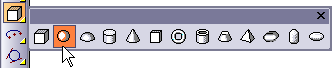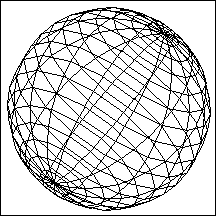# SphereMenu: Solids / SphereToolbox Icon:Draws a solid sphere.

Point 1: Center of the sphere

Point 2: Point on surface of the sphereSpecify the number of sides or facets around both the longitude and latitude of the sphere in the window. The more facets the sphere has, the more spherical it appears.

The first point is the center of the sphere. The second point determines the radius of the sphere. This point can lie in one of three places: Pole, Vertex, or Midpoint.

Vertex: The equator of the sphere is inscribed by a circle of that radius. (The second point lies on the equator at one of the longitudinal divisions.)

Midpoint: The equator of the sphere circumscribes a circle of that radius. (The second point will be on the equator midway between two longitudinal lines.)

Pole: The second point is at the pole and the axis lies along the line between Points 1 and 2